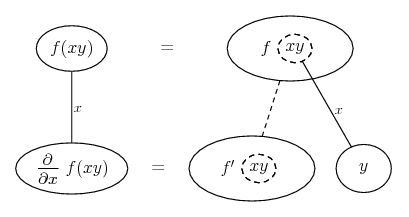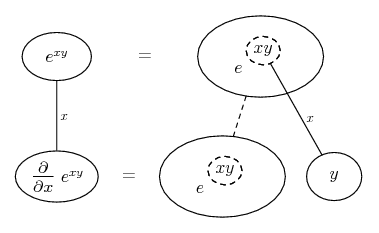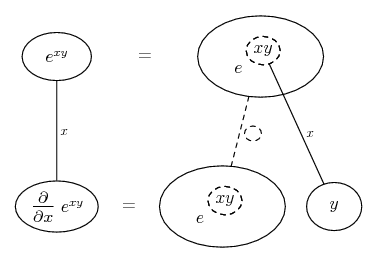# Thread: Partial derivative of a function

1. ## Partial derivative of a function

What is $\displaystyle \frac{\partial}{\partial x}f(xy)$?

I've tried experimenting but I don't know how to simplify it!! Can someone please tell me where to go?

THANKS2.Originally Posted by DivideBy0What is $\displaystyle \frac{\partial}{\partial x}f(xy)$?

I've tried experimenting but I don't know how to simplify it!! Can someone please tell me where to go?

THANKS$\displaystyle \frac{\partial}{\partial x} \left( f( g(x,y) \right) = f_x( \, g(x,y) \, ) \cdot \frac{\partial}{\partial x} ( \, g(x,y) \, )$.

This comes from the corresponding single variable fomrula:
$\displaystyle ( \,f(g(x)) \, )'=f'(g(x)) \cdot g'(x)$.

3.Originally Posted by Miss$\displaystyle \frac{\partial}{\partial x} \left( f( g(x,y) \right) = f_x( \, g(x,y) \, ) \cdot \frac{\partial}{\partial x} (xy)$.

This comes from the corresponding single variable fomrula:
$\displaystyle ( \,f(g(x)) \, )'=f'(g(x)) \cdot g'(x)$.
It's a bit confusing to use g(x,y) and then just xy for the last part. Of course, you mean the chain rule,
$\displaystyle \frac{\partial f(g(x,y)}{\partial x}= \frac{df}{dg}\frac{\partial xy}{\partial x}$

Here, g(x,y)= xy so $\displaystyle \frac{\partial f(xy)}{\partial x}= f '(xy) \frac{\partial xy}{\partial x}= yf '(xy)$
where the f ' is the ordinary derivative of f thought of as a function of a single variable.

4. Hold on, are you saying that

$\displaystyle \frac{\partial}{\partial x}f(xy) = y\frac{\partial}{\partial x}f(xy)$?

This is what I also got when I tried it, but it can't be right, can it?

5.Originally Posted by DivideBy0Hold on, are you saying that

$\displaystyle \frac{\partial}{\partial x}f(xy) = y\frac{\partial}{\partial x}f(xy)$?

This is what I also got when I tried it, but it can't be right, can it?
Why do you think it is not right?

6. Just in case a picture helps...... where... is the chain rule. Straight continuous lines differentiate downwards (integrate up) with respect to x (in the single variable case we would take this as read but here we label the line just to be clear), and the straight dashed line similarly but with respect to the dashed balloon expression (the inner function of the composite which is subject to the chain rule).

Hope this helps, or doesn't further confuse...
_________________________________________

Don't integrate - balloontegrate!

Balloon Calculus; standard integrals, derivatives and methods

Balloon Calculus Drawing with LaTeX and Asymptote!

7. Thanks for the diagrams Tom, I think I understand how the chain rule works

However my main problem is that if

$\displaystyle \frac{\partial}{\partial x} f(xy) = y\frac{\partial}{\partial x} f(xy)$, then
that is a recursive relationship!

So

$\displaystyle (y-1)\frac{\partial}{\partial x}f(xy) = 0$

So $\displaystyle y=0$?

I don't see why this should follow. And indeed I don't want it to have to follow.

Moreover, say you have $\displaystyle f(xy) = e^{xy}$, then

$\displaystyle \frac{\partial}{\partial x} f(xy) = ye^{xy}$

Clearly $\displaystyle \frac{\partial}{\partial x}f(xy) \neq y\frac{\partial}{\partial x}f(xy)$ unless $\displaystyle y=0$, which I don't want to assume.

There must be a solution that doesn't have $\displaystyle y=0$ is there?

Thanks again

8.Originally Posted by DivideBy0Thanks for the diagrams Tom, I think I understand how the chain rule works

However my main problem is that if

$\displaystyle \frac{\partial}{\partial x} f(xy) = y\frac{\partial}{\partial x} f(xy)$, then
that is a recursive relationship!
Sorry I didn't notice you'd written

$\displaystyle \frac{\partial}{\partial x} f(xy) = y\frac{\partial}{\partial x} f(xy)$

before - which should be

$\displaystyle \frac{\partial}{\partial x} f(xy) = y\frac{\partial}{\partial (xy)} f(xy)$

You could mark the distinction with a u-sub (for xy) or notice that the diagram says we differentiate down the dashed line w.r.t. the dashed balloon expression.

e.g... (good example)Edit:

We could also label the dashed line to show what it's w.r.t...... although this is always the case.

9. thanks again tom,

I think I understand it now#### Search Tags

derivative, function, partial